Include Top

# Introduction: Simulation and Optimization to Determine Optimal Magazine Production Quantity to Maximize Profit

This is an example of DiscoverSim simulation and optimization to determine optimal magazine production quantity to maximize net profit.

Monthly Demand is modeled using a discrete custom distribution.

A DiscoverSim Input Control is used to set the number of magazines produced (x 10,000).

Production and disposal costs are variable costs per unit. (Note: content and fixed printing costs are covered by advertising and subscriptions).

Revenue = MIN(Monthly_Demand,Monthly_Demand,Magazines_Produced)*Selling_Price_to_Retailers

Total Production Cost = Magazines_Produced*Production_Cost

Total Disposal Cost = IF((Magazines_Produced-Monthly_Demand)>0,Magazines_Produced-Monthly_Demand,0)*Disposal_Cost

Net Profit = Revenue - (Total_Production_Cost + Total_Disposal_Cost)Summary of DiscoverSim Features Demonstrated in Case Study 2:

• Create Input Distributions with Custom Discrete
• Create Discrete Input Controls
• Run Simulation
• Run Optimization

1. Open the workbook Magazine Production – Optimization. A custom discrete input distribution will simulate the variability in monthly demand and is specified in cell C15. The demand quantity data for this custom distribution is given in cells F15: F24 with weights specified in cells G15:G24. The weights are converted to calculated probabilities in cells H15:H24. A DiscoverSim Input Control (Discrete) will be used to specify the magazine production quantity (10,000 to 100,000) at cell C17. The output, Net Profit will be specified at cell C27.

2.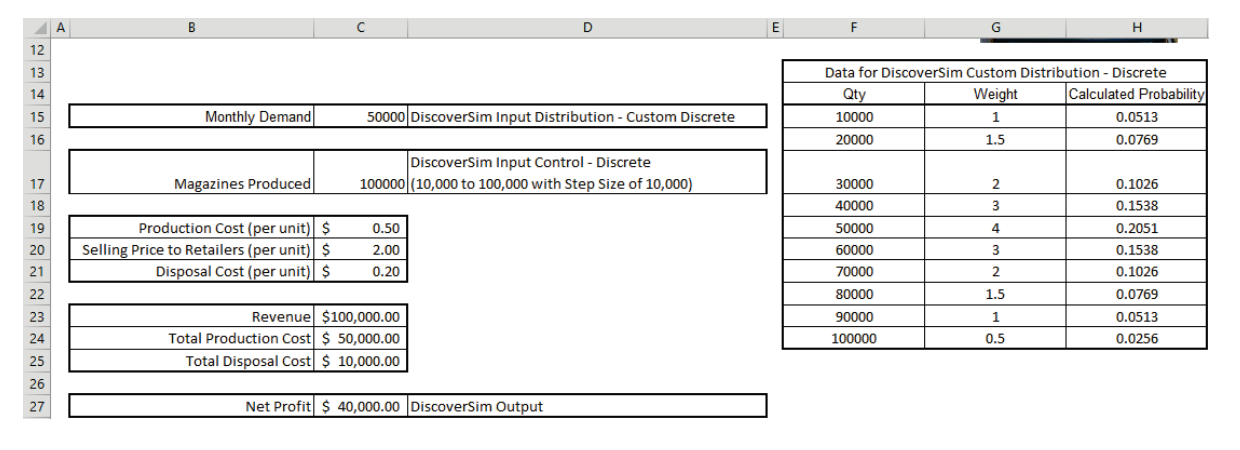3. Click on cell C15 to specify the “Monthly Demand” discrete custom input distribution. Select DiscoverSim > Input Distribution: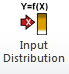4. Select Discrete > Custom Discrete Distribution.

5. Click the input name cell reference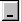and specify cell B15 containing the input Name “Monthly Demand” (Note: spaces are replaced with the underscore “_” character for all input names). After specifying a cell reference, the dropdown symbol changes fromto.

6. Click the Data Range cell referenceand specify cells F15:F24 as the data range for the custom discrete distribution.

7. Click the checkbook to enable Weight Range cell referenceand specify cells G15:G24 as the weight range for the custom discrete distribution. These weights are used to determine the distribution probabilities as displayed in cells H15:H24.

8. Click Update Chart to view the custom discrete distribution as shown:

9.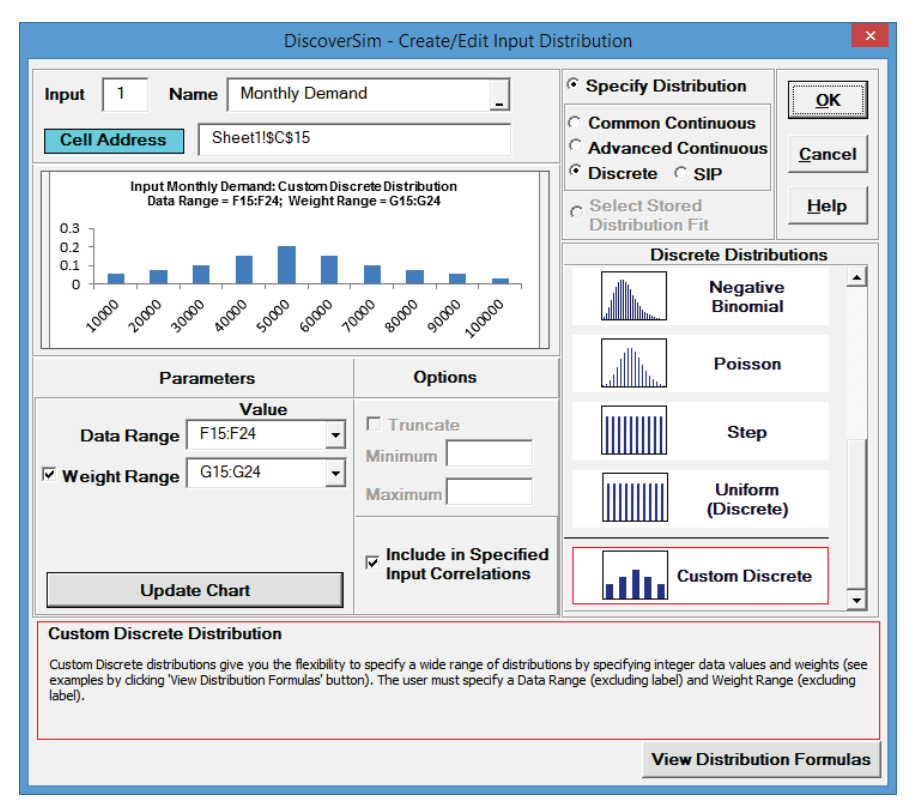10. Click OK. Hover the cursor on cell C15 to view the DiscoverSim graphical comment showing the distribution and parameter values:

11.12. Now we will add the Discrete Input Control (also referred to as a “Decision Variable”). This will be used to set the magazine production quantity (multiplied by 10,000). DiscoverSim will use Stochastic Global Optimization to determine what quantity will maximize the mean net profit.

13. Click on cell C17. Select Control:14. Click input Name cell referenceand specify cell B17 containing the Input Control name “Magazines Produced”.

15. Select Discrete (this Input Control setting will consider only discrete values). The Start value is set to 100000 from cell C17 (current production volume per month). Set the Min value to 10000, Max value to 100000 and Step to 10000 as shown: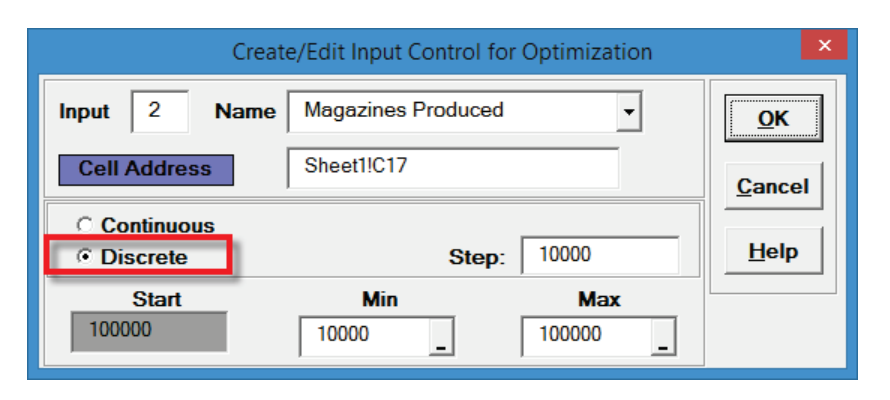16. Click OK. Hover the cursor on cell C17 to view the comment displaying the Input Control settings:

17.18. Now we will specify the output. Click on cell C27. The cell contains the Excel formula for the Net Profit: = _Revenue - (_Total_Production_Cost + _Total_Disposal_Cost).

19. Select DiscoverSim > Output Response:

20.21. Click output Name cell reference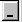and specify cell B27 containing the Output name “Net Profit”. Enter the Lower Specification Limit (LSL) as 0. The Weight and Output Goal settings are used only for multiple response optimization, so do not need to be modified in this example. Click OK.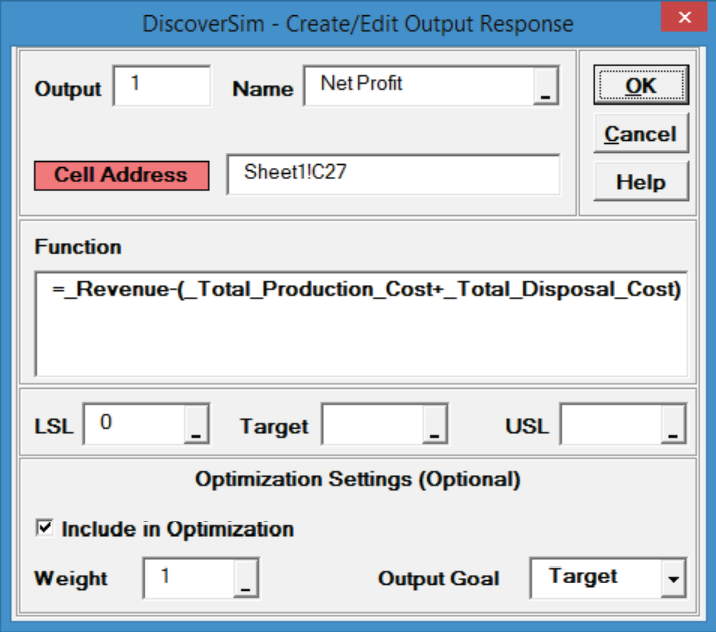22. Hover the cursor on cell C27 to view the DiscoverSim Output information.

23.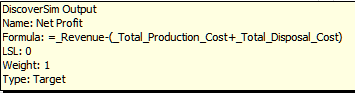24. Select DiscoverSim > Run Simulation:25. Select Seed Value and enter “12” as shown, in order to replicate the simulation results given below  (note that 64 bit DiscoverSim will show slightly different results).Click Run.

26. The DiscoverSim Output Report displays a histogram, descriptive statistics and process capability indices:

27.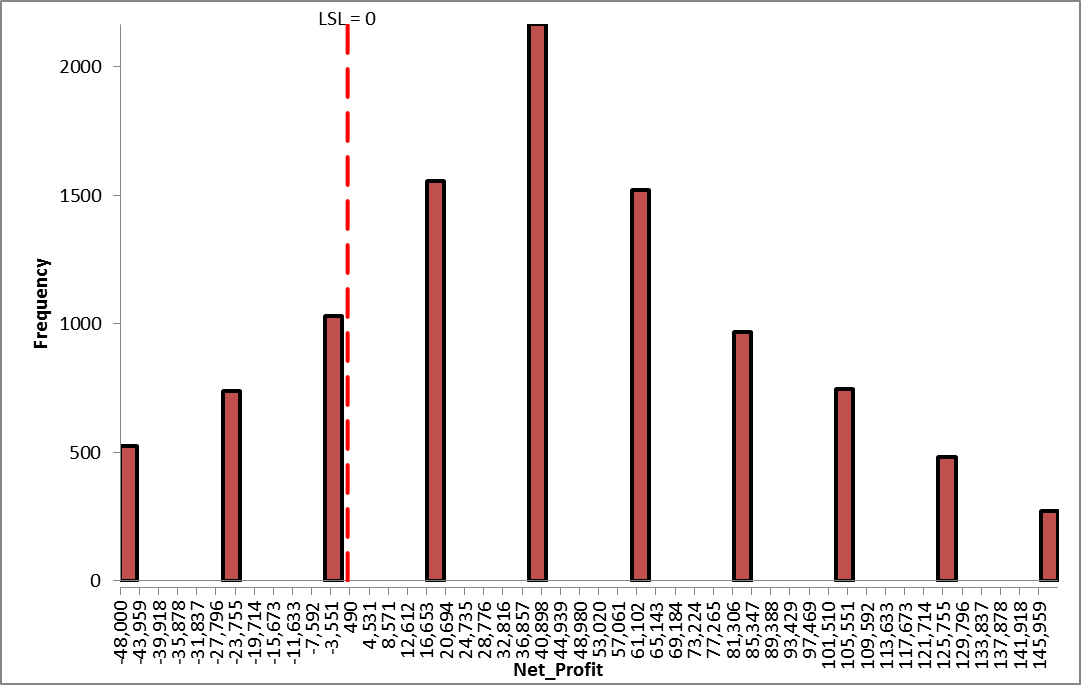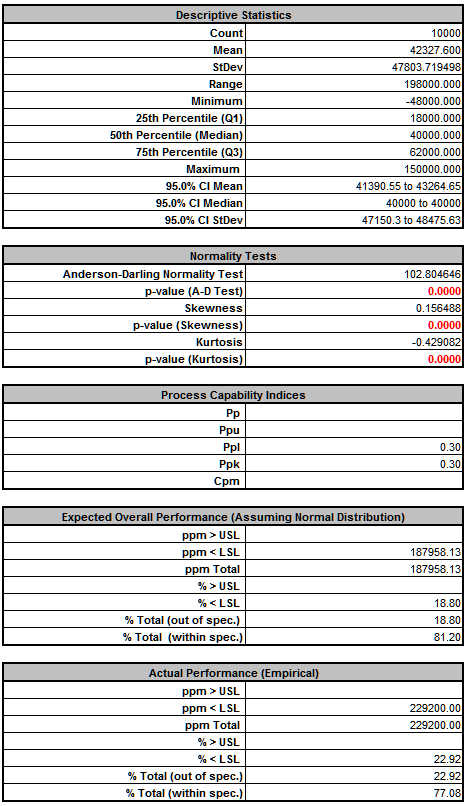The Mean Net Profit is approximately \$42,300, which is good, but we also see from the Actual Performance report, we have a 22.9% chance of monthly loss. The standard deviation is also quite high at \$47,800.

28. Now we are ready to perform the discrete optimization to maximize the mean of net profit. Select DiscoverSim > Run Optimization:

29.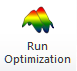30. Select “Maximize” for Optimization Goal, “Weighted Sum” for Multiple Output Metric and “Mean” for Statistic. Select Discrete Exhaustive. Set Seed Value to 12 and Replications to 2500 in order to replicate the optimization results given below. All other settings will be the defaults as shown: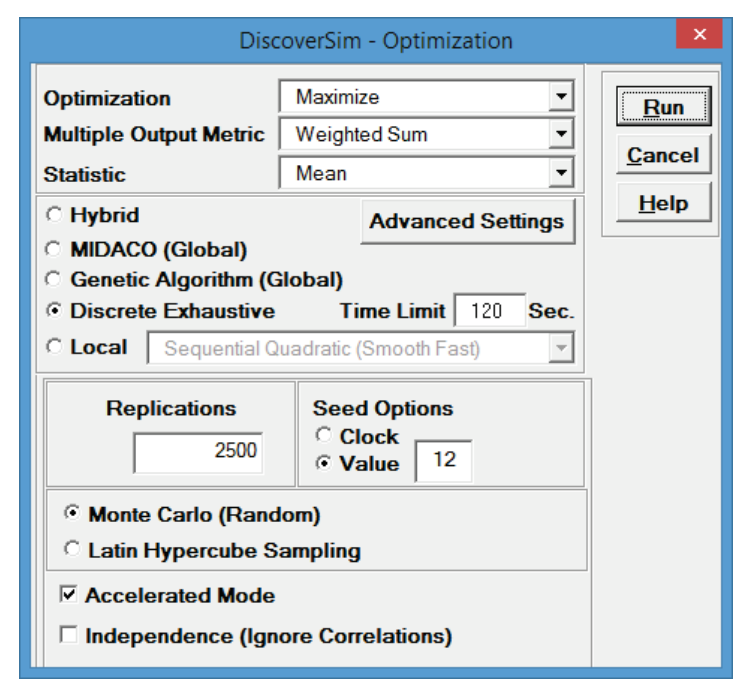Tip: Here we are using Discrete Exhaustive because this particular problem is not complex, given that there is only one input control variable with 10 discrete levels. The Time Limit determines how long Discrete Exhaustive will run. A single simulation run is first performed and the computation time is measured. If the total number of discrete combinations * single run time exceed the Time Limit, an error message is produced, so you can either increase the Time Limit, or use a different optimization method.

31. Click Run.

32. The final optimal parameter value is given as:

33.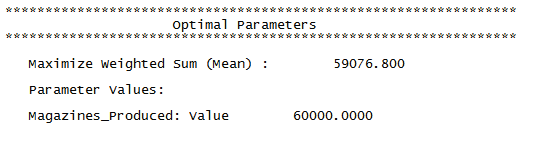The optimum number of magazines to produce per month is 60,000, resulting in an expected mean profit of \$59,077.

34. You are prompted to paste the optimal value into the spreadsheet:

35.Tip: If you check Display History, the complete history of optimization iterations will be displayed in a separate window after clicking Yes or No to the paste prompt.

Click Yes. This sets the input control value to the optimum value of 6 as shown:36. Select DiscoverSim > Run Simulation:37. Select Seed Value and enter “12” as shown, in order to replicate the simulation results given below.

38.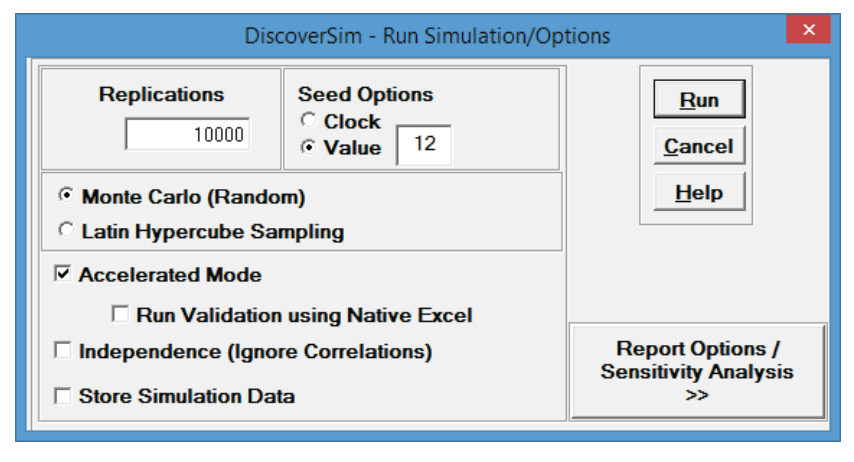Click Run.

39. The DiscoverSim Output Report displays a histogram, descriptive statistics and process capability indices for the optimized setting:

40.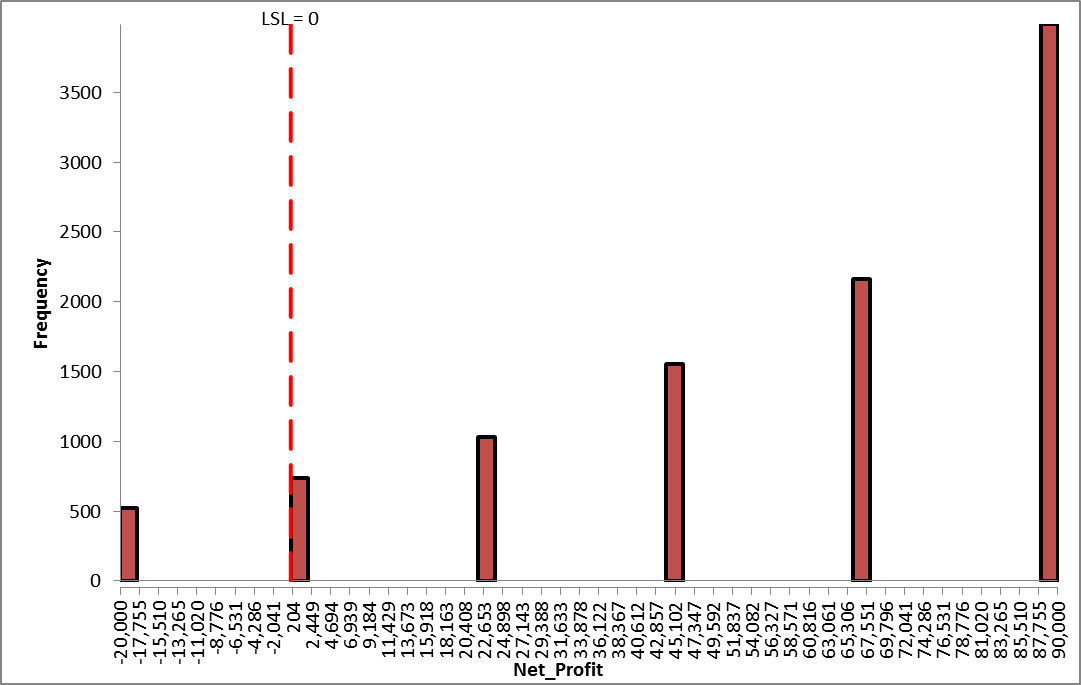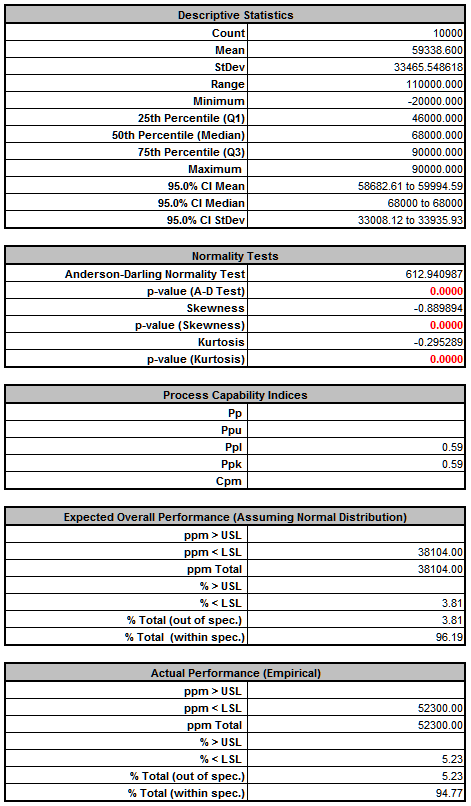From the histogram and capability report we see that the expected mean net profit has increased from \$42,300 to \$59,300. The standard deviation has decreased from \$47,800 to \$33,500. Looking at the Actual Performance report we see a decrease in the likelihood of a profit loss from 22.9% to 5.2%.

41. Obviously the quality of this prediction will depend on the validity of the custom distribution and does not take into account hidden soft costs like customer dissatisfaction due to lack of availability. If necessary, the model can be refined. Nevertheless, the tool is very useful to help us to determine what our production rate should be in order to maximize net profit.

# Web Demos

Our CTO and Co-Founder, John Noguera, regularly hosts free Web Demos featuring SigmaXL and DiscoverSim